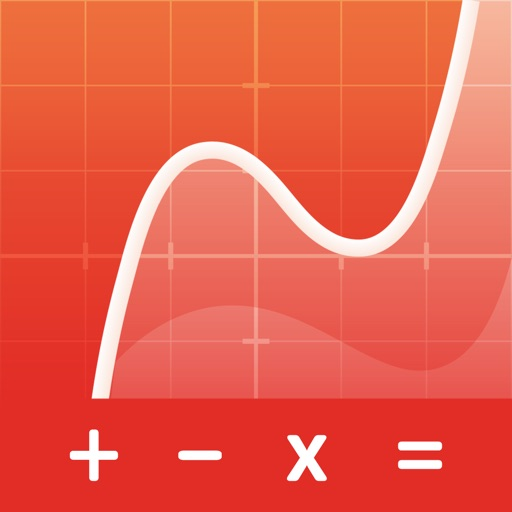Graphing Calculator Pro²50% OFF# Graphing Calculator Pro²

## Scientific Graphing Calculator

\$4.99 · \$9.99
7.3

978 Ratings

Size

37.2 MB

### Screenshots for iPhone

iPhone
Graphing Calculator Pro² Description
A full-featured graphing calculator: Graph and investigate functions, solve equations, work with statistics, distributions, matrices and much more.

FIVE REASONS WHY YOU NEED THIS APP
1. Keyboard and command syntax similar to physical graphing calculators -> No need to learn a new calculator.
2. Powerful emulator like Wabbitemu Calculator -> No more waiting to graph functions compared to slow physical handhelds.
3. Enter fractions, equations or functions in the same way as you would write them on paper.
5. High-resolution graphs

FULL GRAPHING CALCULATOR
• Graph more than ten functions at once, each with a different color
• Add Lines, Horizontals, Verticals, and Tangents to the graph
• Use your finger or the arrow keys to trace the coordinates of your functions
• Calculate the Zero, Minimum, Maximum, Intersection, and Derivative
• Find integrals and color the area under the function
• Set the X and Y coordinates of the graphing window; zoom in and out with your fingers

• Enter calculations in natural textbook format
• Includes all basic arithmetic (+, -, x, ÷, ^, ², √, π, …)
• Use advanced functions like sin, cos, tan, e, ln, Σ, ...
• Scroll your calculation history and modify existing calculations with one tab
• Store and recall variables

MATRIX CALCULATOR
• Work with matrices the easy way -> store them or enter them directly on the calculator interface
• Do basic matrix arithmetic operations like addition, subtraction, multiplication or power of a Matrix
• Put the matrix into Reduced Row-Echelon Form and solve linear equation systems
• Calculate the determinant, inversion or transpose the matrix

STATISTICS AND LISTS
• Use lists for all basic and trigonometric ​operations
• Enter lists using the Statistics List Editor or use curly brackets on the main screen
• Perform 1- and 2- Variable Stats
• Investigate your lists: min, max, mean, median, sum, product, standard deviation, and variance.​
• Do regressions with best-fit lines: LinReg, QuadReg, CubicReg, QuartReg, ExpReg
• Work with distributions: Normal, Poisson and Binomial

TUTORIAL AND CATALOG
• Easy to understand tutorial to get started
• Full Catalog of all commands with syntax, example, and possible error messages
• Tab on highlighted math terms to read on point definitions

PERFECT FOR HIGH SCHOOL & COLLEGE STUDENTS
• Recommended by teachers to use in math classes
• Pre-Algebra, Algebra 1 & 2
• Pre-Calculus, Calculus
• Statistics
• Permitted on SAT/ ACT/ AP (your phone might not be permitted, but you can use the app for homework and borrow an actual calculator from your school for the test)
Graphing Calculator Pro² 1.4.3 Update
2020-12-18 Version History
+ Fixed a bug that table didn't work.
+ New side menu that makes it easier for you to quickly access links. Open it by swiping left to right in the calculations menu.
+ Support for piecewise functions.

New commands are available!
+ Med-Med
+ randNorm
+ randBin
+ Matrix-List
+ List-Matrix
+ DrawInv
+ 2-PropZ-Interval
+ Chi²-Test
+ Chi²GOF-Test
+ LinRegT-Test
+ LinRegT-Interval
+ ANOVA
More